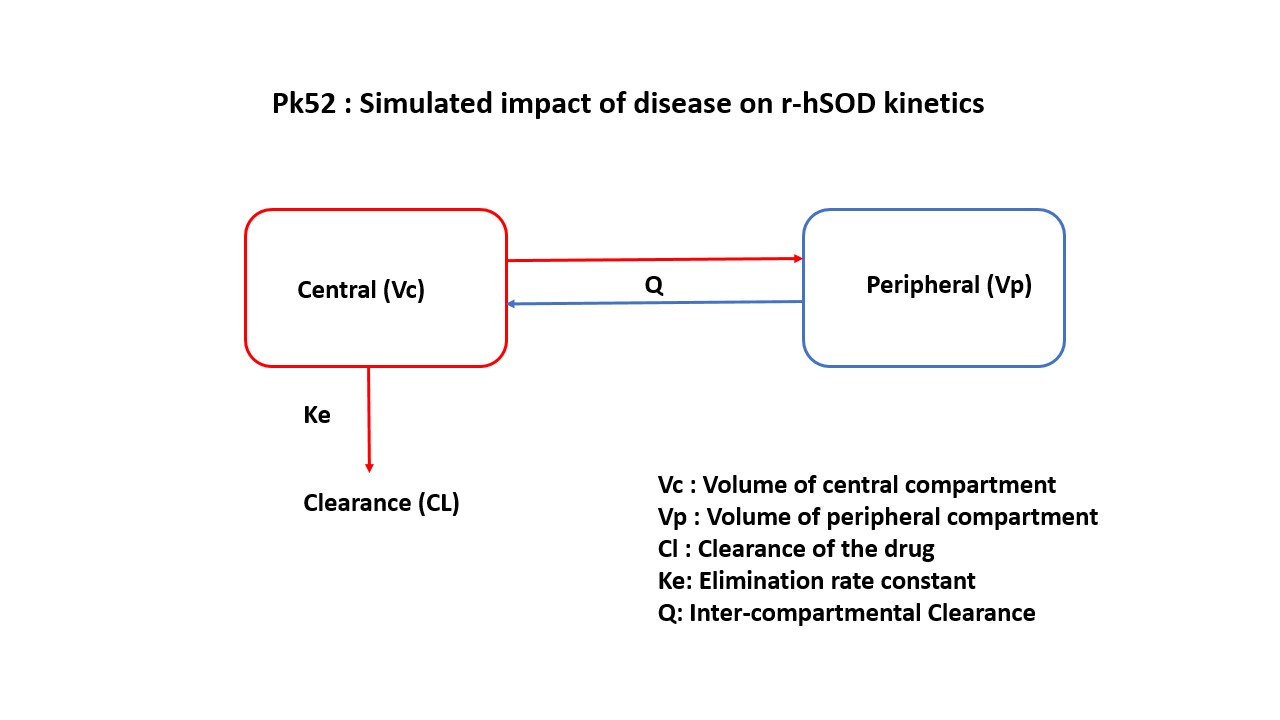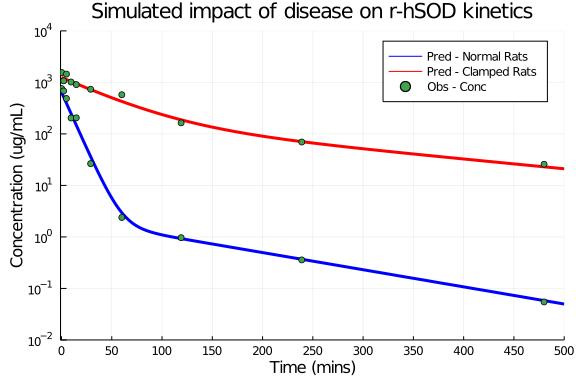# Exercise PK52 - Simulated impact of disease on r-hSOD kinetics

### Background

• Structural model - IV infusion - Two compartment Model

• Route of administration - Rapid intravenous infusion of recombinant human superoxide dismutase (r-hSOD)

• Dosage Regimen - 20mg/kg IV rapid infusion for 15 seconds in two categories of rats

• Number of Subjects - 1 normal rate, 1 clamped (nephrectomized) ratIn this model, collection of plasma concentration data of parent drug and concentration of parent and metabolite in urine, will help you to derive/ estimate the parameters: Clearance, Volume of Distribution, Km, Vmax.

### Learning Outcome

1. To note that clearance and volume of central compartment are reduced in clamed rats, as removal of the kidneys increased the effective half life from 10mins in normal rats to 90mins in nephrectomized rats.

2. The time to steady state might differ 9-fold between the two groups.

3. Importance of kidneys in the elimination of r-hSOD and the impact of kidney disease/nephrectomy in the overall kinetics of the drug.

### Objectives

In this tutorial, you will learn how to construct a simple two compartment model with 2 set of parameters corresponding to normal and clamped rats.

### Libraries

call the "necessary" libraries to get started

using Pumas
using Plots
using CSV
using StatsPlots
using Random


### Model

In this two compartmental model, we administer dose on Central compartment.

pk_52           = @model begin
@param begin
tvcl       ∈ RealDomain(lower=0)
tvvc       ∈ RealDomain(lower=0)
tvvp       ∈ RealDomain(lower=0)
tvcld      ∈ RealDomain(lower=0)
Ω          ∈ PDiagDomain(4)
σ²_prop    ∈ RealDomain(lower=0)
end

@random begin
η           ~ MvNormal(Ω)
end

@pre begin
Cl          = tvcl * exp(η)
Vc          = tvvc * exp(η)
Vp          = tvvp * exp(η)
Cld         = tvcld * exp(η)
end

@dynamics begin
Central'    = -(Cl/Vc)*Central - (Cld/Vc)*Central + (Cld/Vp) * Peripheral
Peripheral' =  (Cld/Vc)*Central - (Cld/Vp)*Peripheral
end

@derived begin
cp          = @. Central/Vc
dv          ~ @. Normal(cp,sqrt(cp^2*σ²_prop))
end
end

PumasModel
Parameters: tvcl, tvvc, tvvp, tvcld, Ω, σ²_prop
Random effects: η
Covariates:
Dynamical variables: Central, Peripheral
Derived: cp, dv
Observed: cp, dv


### Parameters

Parameters are provided for the simulation as below. tv represents the typical value for parameters.

• tvcl - Clearance (mL/min/kg)

• tvvc - Volume of Central Compartment (ml/min/kg)

• tvvp - Volume of Peripheral CompartmentRenal (ml/min/kg)

• tq - Intercompartmental Clearance (ml/min/kg)

• Ω - Between Subject Variability

• σ - Residual errors

param1 = (tvcl    = 3.0,
tvvc    = 31,
tvvp    = 15,
tvcld   = 0.12,
Ω       = Diagonal([0.0,0.0,0.0,0.0]),
σ²_prop = 0.04)

param2 = (tvcl    = 0.22,
tvvc    = 16,
tvvp    = 13,
tvcld   = 0.09,
Ω       = Diagonal([0.0,0.0,0.0,0.0]),
σ²_prop = 0.04)

param  = vcat(param1, param2)


### Dosage Regimen

A dose of 20 mg/kg to a single rat of 2 categories (normal and clamped)

ev1      = DosageRegimen(20000,cmt=1)
sub1     = Subject(id=1, events=ev1, covariates=(Rat="Normal_Rat",))
sub2     = Subject(id=2, events=ev1, covariates=(Rat="Clamped_Rat",))
pop2_sub = [sub1,sub2]

Population
Subjects: 2
Covariates: Rat


### Simulation

Simulate the plasma concentration after IV infusion

Random.seed!(123)
sim_pop2_sub = map(((subject, param),) -> simobs(pk_52, subject, param, obstimes=0:0.1:500), zip(pop2_sub, param))
df           = DataFrame(sim_pop2_sub)


### Dataframe & Plot

Use the dataframe for plotting

• cp - Predicted concentration

• dv - Observed concentration

df1 = filter(x -> x.id == "1", df)
df2 = filter(x -> x.id == "2", df)
df_dv = filter(x-> x.time in [0.2,2.1,4.9,9.5,14.7,29,60,119,239,480],df)

@df df1 plot(:time, :cp, yaxis=:log,
title="Simulated impact of disease on r-hSOD kinetics", label= "Pred - Normal Rats",
xlabel= "Time (mins)", ylabel="Concentration (ug/mL)", color=[:blue], linewidth=3,
xlims=(-1,500), xticks=[0,50,100,150,200,250,300,350,400,450,500], yticks=[0.01,0.1,1,10,100,1000,10000], ylims=(0.01,10000))
@df df2 plot!(:time, :cp,linewidth=3, label= "Pred - Clamped Rats", color=[:red])
@df df_dv scatter!(:time, :dv, label = "Obs - Conc")